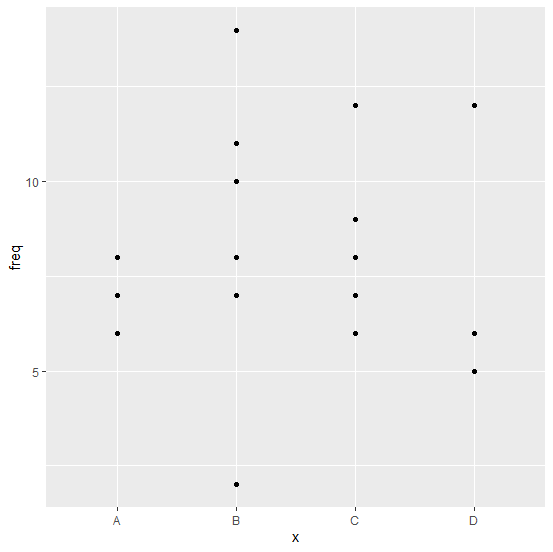# How to create a point chart for categorical variable in R?

The point chart of a categorical has points corresponding to the categories just like the bar chart has bars. If we want to create point chart for categorical variable then we just need to use geom_point function of ggplot2 package. For example, if we have a data frame df that contains categorical column x and frequency column defined sd freq then the point chart for the categories in x can be ggplot(df,aes(x,freq))+geom_point().

## Example

Consider the below data frame:

Live Demo

> set.seed(3521)
> x<-sample(LETTERS[1:4],20,replace=TRUE)
> freq<-rpois(20,8)
> df<-data.frame(x,freq)
> df

## Output

x freq
1 B 2
2 C 12
3 A 8
4 D 12
5 C 8
6 C 9
7 B 11
8 B 10
9 B 8
10 C 6
11 D 5
12 B 10
13 D 6
14 B 7
15 B 14
16 C 7
17 A 7
18 A 6
19 A 6
20 A 7

> library(ggplot2)
> ggplot(df,aes(x,freq))+geom_point()